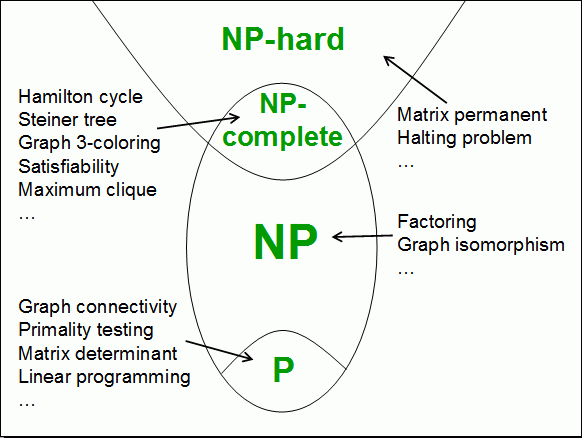# What Is NP CompleteAllPages RecentChanges Links to this page Edit this page Search Entry portal Advice For New Users

Prev: Some Example NP Problems / Next: How do you prove a problem is NPC?

 This is just re-stating what it means to say that problem A is harder than problem B.
A problem H is "NP-Hard" if it's at least as hard as every problem from NP. That means that if you give me an instance, any instance, from a problem, any problem, from NP, then I can convert it into an instance of H such that a solution to that instance can be converted back to a solution of the original instance.More specifically, consider Graph Three-Colouring (G3C), and suppose I claim that G3C is NP-Hard. Now you come along with a problem from NP. Perhaps you're interested in integer factorisation. My claim that G3C is NP-Hard means that whenever you have a number n to factor, I can create a graph such that three-colouring that graph lets me return to you a factor of n.

Sounds unlikely? Perhaps, but it turns out to be true, and is used as the example in the next section.

But to show that a problem is NP-Hard we must show that this can be done for every NP problem. We must show that H is at least as hard as every problem from NP. That's rather a tall order, but we'll see how that works shortly.

But that's NP-Hard. What's NP-Complete?

A problem is NP-Complete if it is both itself in NP and is also NP-Hard. In other words, it's (among) the hardest of the problems in NPC.

 Notice that this sort-of assumes that there are problems in NP that aren't in P. If not, the whole thing collapses. And that's the point of the "P vs NP" problem.
So now we have an overview of this small part of the complexity zoo.

• NP-Hard means at least as hard as everything in NP.
• There are problems in the intersection of NP-Hard and NP.
• These are called NP-Complete.
• NP problems contain all P problems
In the next section we'll see how a problem can be proven to be NP-Complete.
Prev: Some Example NP Problems / Next: How do you prove a problem is NPC?